# Principles of Microeconomics 13 Industrial Organization and Welfare

• Slides: 38Principles of Microeconomics 13. Industrial Organization and Welfare* Akos Lada August 8 th, 2011 * Slide content principally sourced from N. Gregory Mankiw “Principles of Economics” Premium Powe. Point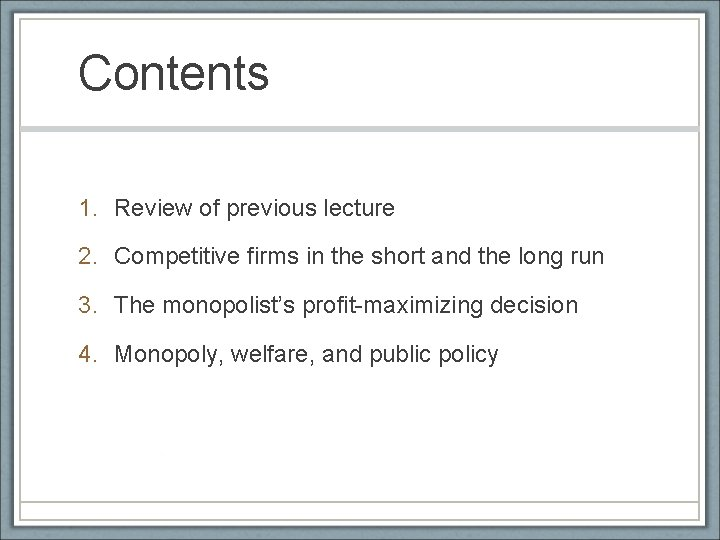Contents 1. Review of previous lecture 2. Competitive firms in the short and the long run 3. The monopolist’s profit-maximizing decision 4. Monopoly, welfare, and public policy1. Review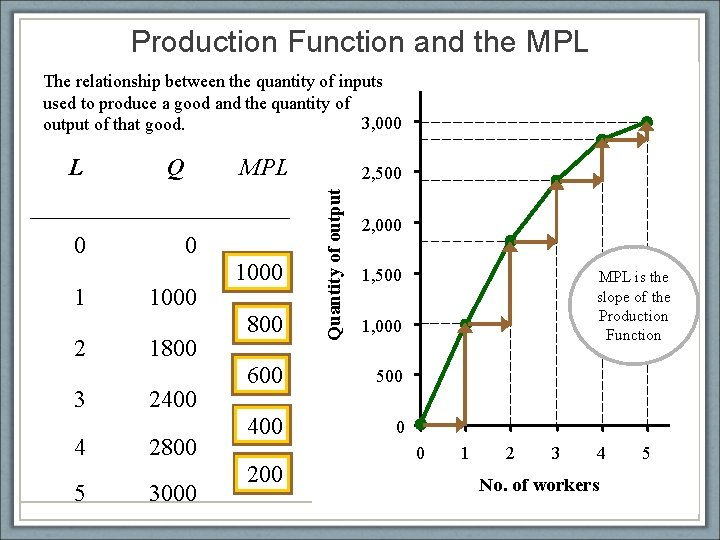Production Function and the MPL The relationship between the quantity of inputs used to produce a good and the quantity of 3, 000 MPL output of that good. 0 1 2 3 4 5 Q MPL 0 1000 1800 2400 2800 3000 1000 800 600 400 200 Quantity of output L equals the slope of the production 2, 500 function. 2, 000 Notice that MPL diminishes 1, 500 as L increases. 1, 000 MPL is the slope of the Production Function This explains why the 500 production function gets flatter 0 as L 0 increases. 1 2 3 4 No. of workers 5Production Costs \$800 FC Q \$700 VC FC VC TC \$100 \$0 \$100 \$600 1 100 70 170 \$500 2 100 120 220 3 100 160 260 4 100 210 310 5 100 280 380 6 100 380 480 7 100 520 620 Costs 0 TC \$400 \$300 \$200 \$100 \$0 0 1 2 3 4 Q 5 6 7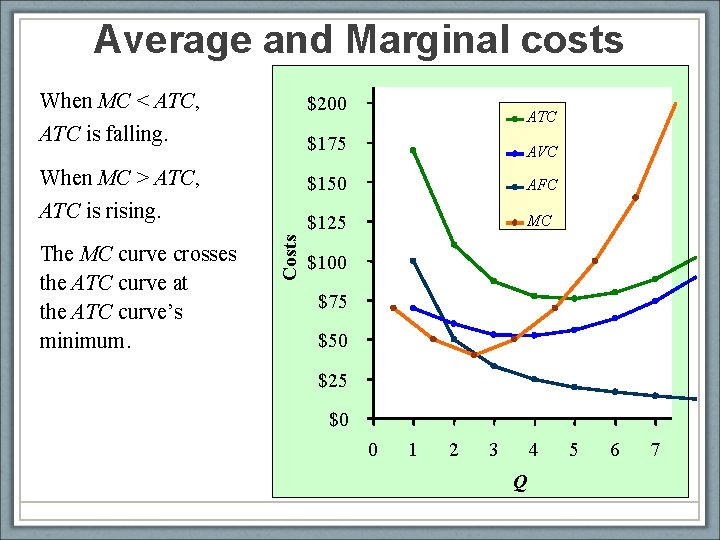Average and Marginal costs When MC < ATC, ATC is falling. \$200 \$175 AVC When MC > ATC, \$150 AFC \$125 MC The MC curve crosses the ATC curve at the ATC curve’s minimum. Costs ATC is rising. ATC \$100 \$75 \$50 \$25 \$0 0 1 2 3 4 Q 5 6 7Refer to the table below. The average total cost of producing five units of output is Quantity of Output 0 1 2 3 4 5 1. \$2. 2. \$5. 3. \$9. 4. \$45. Fixed Costs \$20 20 20 Variable Costs \$0 5 10 15 20 25Refer to the table below. The marginal cost of producing the fifth unit of output is Quantity of Output 0 1 2 3 4 5 1. \$8. 2. \$18. 3. \$40. 4. \$58. Fixed Costs \$10 10 10 Variable Costs \$0 4 9 15 22 40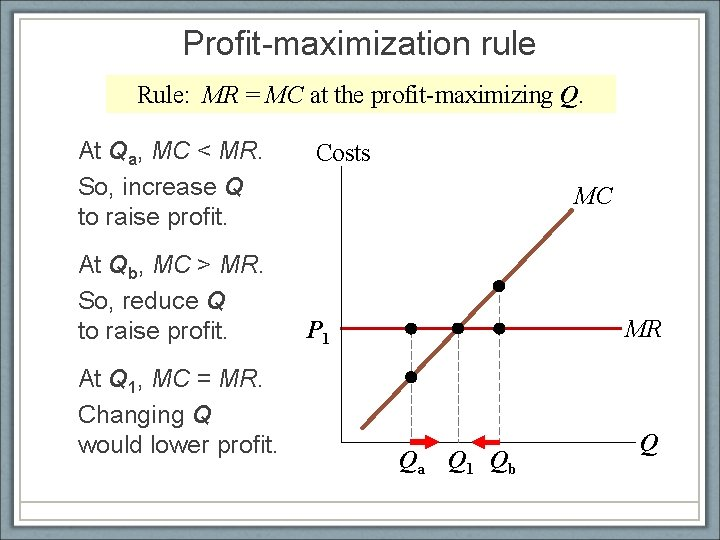Profit-maximization rule Rule: MR = MC at the profit-maximizing Q. At Qa, MC < MR. So, increase Q to raise profit. At Qb, MC > MR. So, reduce Q to raise profit. At Q 1, MC = MR. Changing Q would lower profit. Costs MC MR P 1 Qa Q 1 Qb Q2. Competitive firms in the short and the long runFirms’ Shutdown and Exit • Shutdown: A short-run decision not to produce anything because of market conditions. • Exit: A long-run decision to leave the market. • A key difference: • If shut down (short run), must still pay FC. • If exit (long run), zero costs.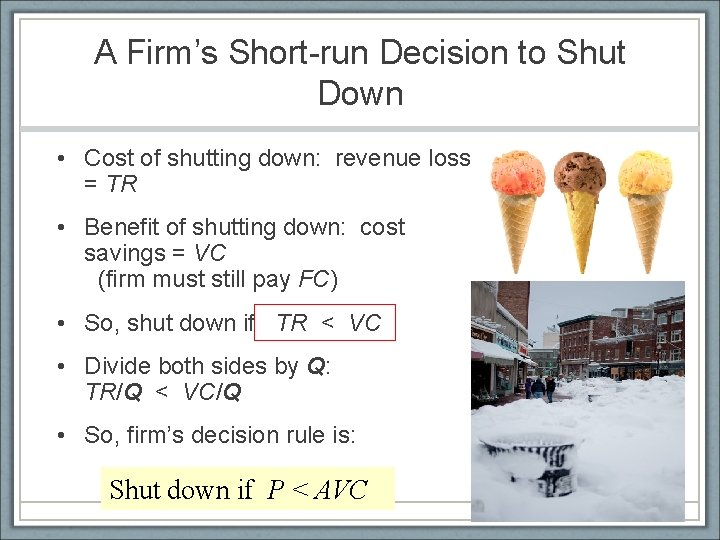A Firm’s Short-run Decision to Shut Down • Cost of shutting down: revenue loss = TR • Benefit of shutting down: cost savings = VC (firm must still pay FC) • So, shut down if TR < VC • Divide both sides by Q: TR/Q < VC/Q • So, firm’s decision rule is: Shut down if P < AVC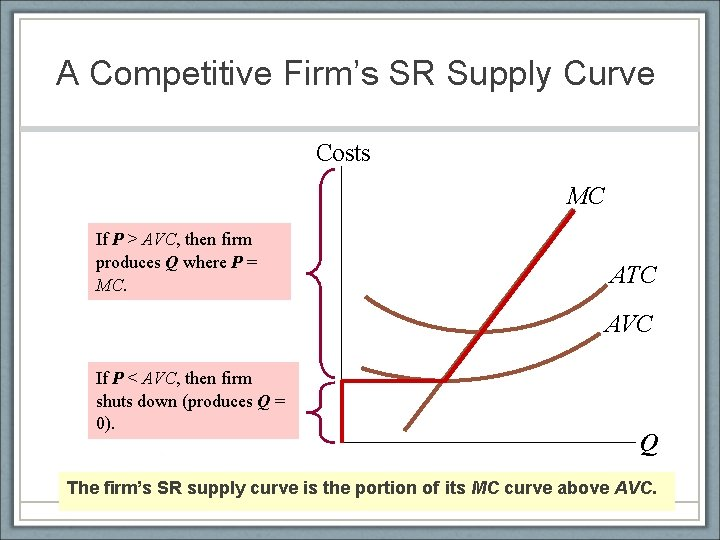A Competitive Firm’s SR Supply Curve Costs MC If P > AVC, then firm produces Q where P = MC. ATC AVC If P < AVC, then firm shuts down (produces Q = 0). Q The firm’s SR supply curve is the portion of its MC curve above AVC.The Chocolate Moose Ice Cream Store is a business that closes from November to April each year. The best explanation for closing during these months is that the store’s 1. revenues are insufficient to cover total costs. 2. total fixed costs are less than marginal fixed cost. 3. revenue per unit is less than average variable cost. 4. marginal costs are less than the revenues.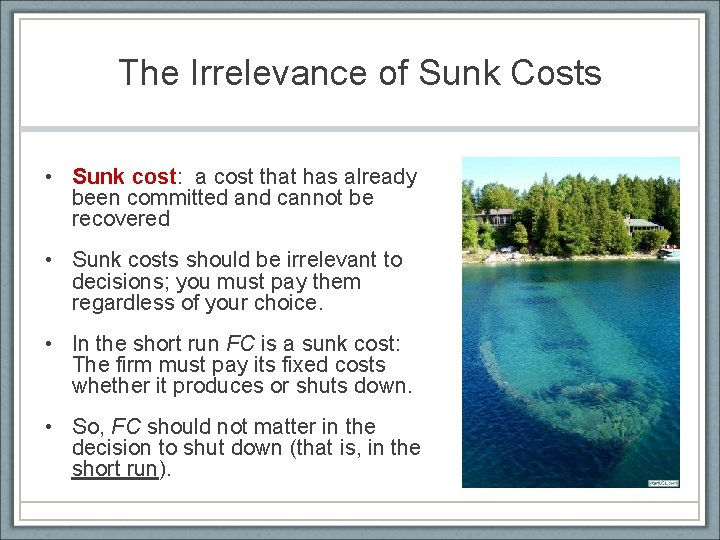The Irrelevance of Sunk Costs • Sunk cost: a cost that has already been committed and cannot be recovered • Sunk costs should be irrelevant to decisions; you must pay them regardless of your choice. • In the short run FC is a sunk cost: The firm must pay its fixed costs whether it produces or shuts down. • So, FC should not matter in the decision to shut down (that is, in the short run).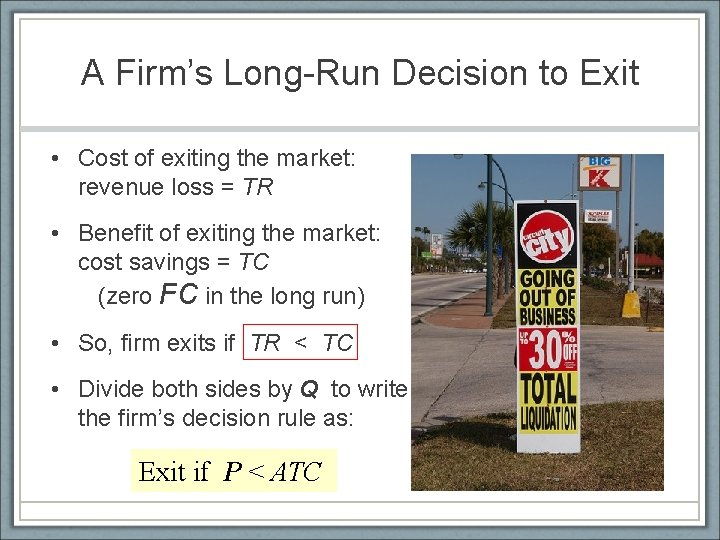A Firm’s Long-Run Decision to Exit • Cost of exiting the market: revenue loss = TR • Benefit of exiting the market: cost savings = TC (zero FC in the long run) • So, firm exits if TR < TC • Divide both sides by Q to write the firm’s decision rule as: Exit if P < ATC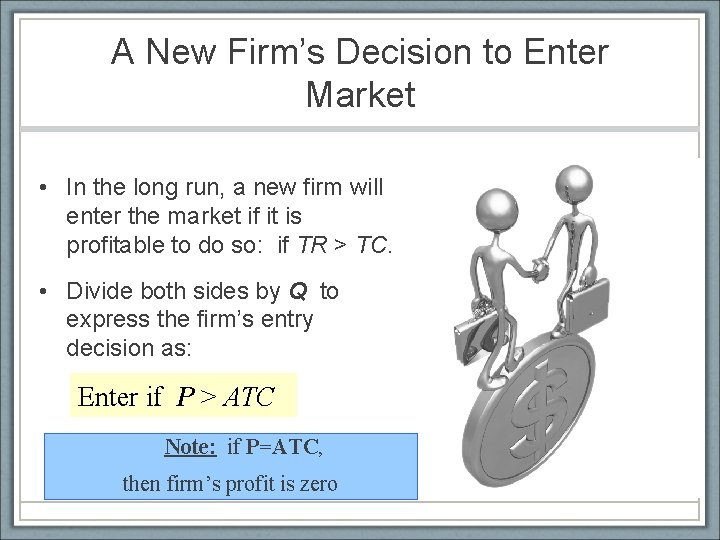A New Firm’s Decision to Enter Market • In the long run, a new firm will enter the market if it is profitable to do so: if TR > TC. • Divide both sides by Q to express the firm’s entry decision as: Enter if P > ATC Note: if P=ATC, then firm’s profit is zero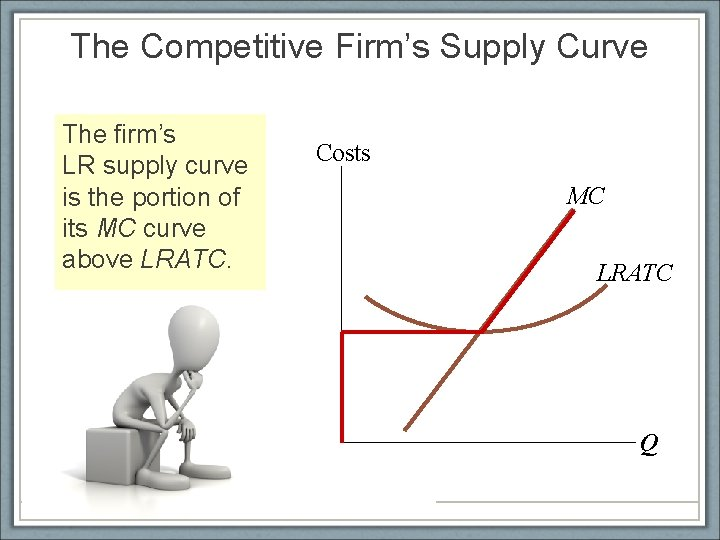The Competitive Firm’s Supply Curve The firm’s LR supply curve is the portion of its MC curve above LRATC. Costs MC LRATC Q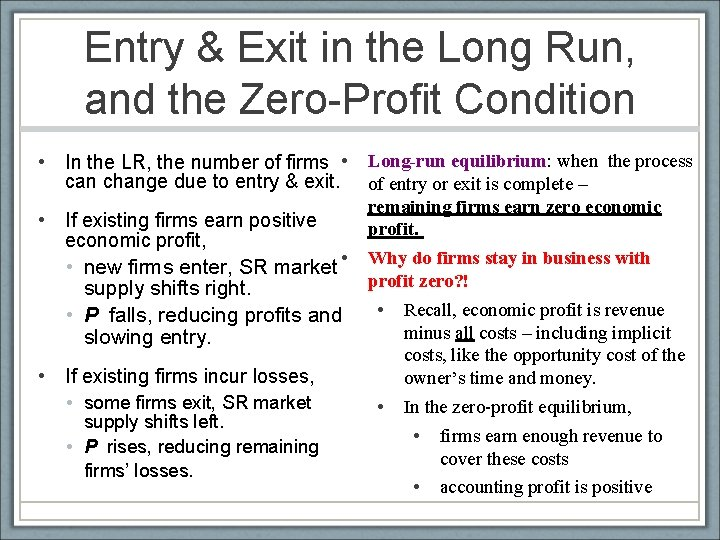Entry & Exit in the Long Run, and the Zero-Profit Condition • In the LR, the number of firms • Long-run equilibrium: when the process can change due to entry & exit. of entry or exit is complete – remaining firms earn zero economic • If existing firms earn positive profit. economic profit, • new firms enter, SR market • Why do firms stay in business with profit zero? ! supply shifts right. • Recall, economic profit is revenue • P falls, reducing profits and minus all costs – including implicit slowing entry. • If existing firms incur losses, • some firms exit, SR market supply shifts left. • P rises, reducing remaining firms’ losses. costs, like the opportunity cost of the owner’s time and money. • In the zero-profit equilibrium, • firms earn enough revenue to cover these costs • accounting profit is positiveSTUDENT’S TURN Identifying a firm’s profit Determine this firm’s total profit. Identify the area on the graph that represents the firm’s profit. Costs, P A competitive firm MC MR ATC P = \$10 \$6 50 Q 20Answers Costs, P Profit per unit = P – ATC = \$10 – 6 = \$4 A competitive firm MC MR ATC P = \$10 profit \$6 Total profit = (P – ATC) x Q = \$4 x 50 = \$200 50 Q 21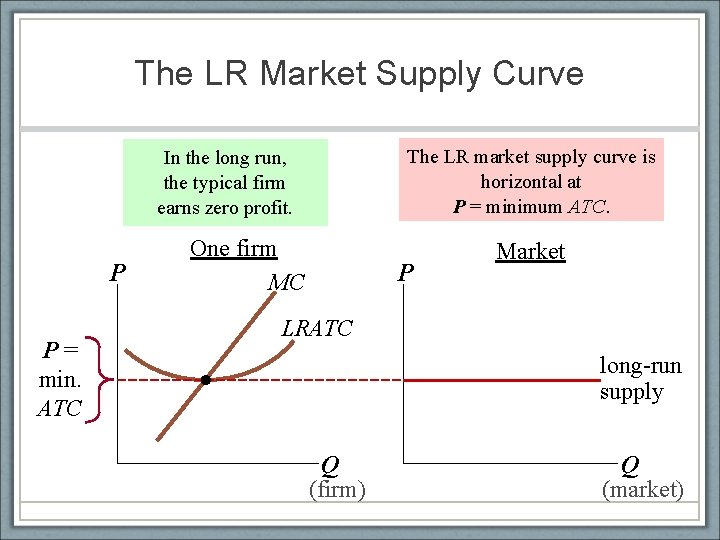The LR Market Supply Curve The LR market supply curve is horizontal at P = minimum ATC. In the long run, the typical firm earns zero profit. P P= min. ATC One firm MC P Market LRATC long-run supply Q (firm) Q (market)SR & LR effects of an Increase in Demand A firm begins in longrun equilibrium… …leading to SR profits for the firm. …but then an increase in demand raises P, … Over time, profits induce entry, shifting S to the right, reducing P… …driving profits to zero and restoring long-run equilibrium. P Market One firm S 1 MC P Profit S 2 ATC B P 2 P 1 A C long-run supply D 2 D 1 Q (firm) Q 1 Q 2 Q 3 Q (market)3. The monopolist’s profitmaximizing decisionA monopoly is different… • For a competitive firm: • At a given quantity, what is the revenue it makes, on average, per each unit sold? • AR = P • If it wants to increase this quantity by one unit, what is the additional revenue it can expect to receive? • MR = P • So: MR=AR=P • For a monopoly: • At a given quantity, what is the revenue it makes, on average, per each unit sold? • AR = P • If it wants to increase this quantity by one unit, what is the additional revenue it can expect to receive? • MR < P !!! • Why? ? ? • Because to be able to sell more, it needs to reduce the price.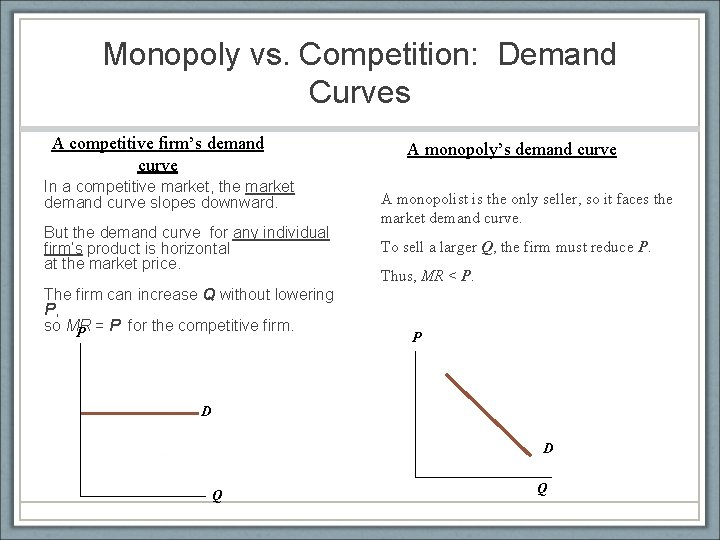Monopoly vs. Competition: Demand Curves A competitive firm’s demand curve In a competitive market, the market demand curve slopes downward. But the demand curve for any individual firm’s product is horizontal at the market price. The firm can increase Q without lowering P, so MR P = P for the competitive firm. A monopoly’s demand curve A monopolist is the only seller, so it faces the market demand curve. To sell a larger Q, the firm must reduce P. Thus, MR < P. P D D Q QUnderstanding the Monopolist’s MR • Increasing Q has two effects on revenue: • Output effect: higher output raises revenue • Price effect: lower price reduces revenue • To sell a larger Q, the monopolist must reduce the price on all the units it sells. • Hence, MR < P • MR could even be negative if the price effect exceeds the output effect!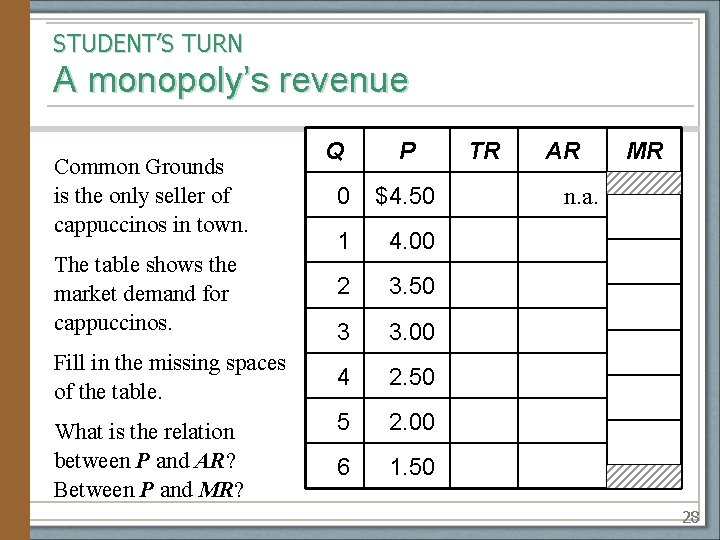STUDENT’S TURN A monopoly’s revenue Common Grounds is the only seller of cappuccinos in town. The table shows the market demand for cappuccinos. Fill in the missing spaces of the table. What is the relation between P and AR? Between P and MR? Q P 0 \$4. 50 1 4. 00 2 3. 50 3 3. 00 4 2. 50 5 2. 00 6 1. 50 TR AR MR n. a. 28Answers Here, P = AR, same as for a competitive firm. Here, MR < P, whereas MR = P for a competitive firm. Q P TR AR 0 \$4. 50 \$0 n. a. 1 4. 00 4 \$4. 00 2 3. 50 7 3. 50 3 3. 00 9 3. 00 4 2. 50 10 2. 50 5 2. 00 10 2. 00 6 1. 50 9 1. 50 MR \$4 3 2 1 0 – 1 29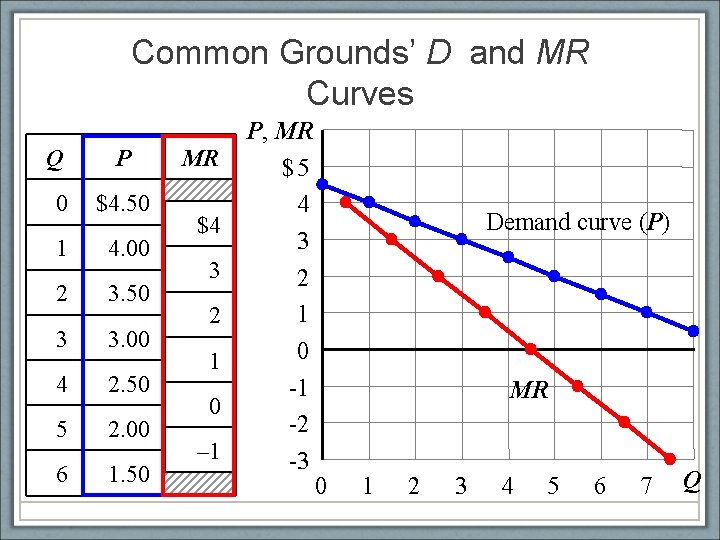Common Grounds’ D and MR Curves Q P 0 \$4. 50 1 4. 00 2 3. 50 3 3. 00 4 2. 50 5 2. 00 6 1. 50 MR \$4 3 2 1 0 – 1 P, MR \$5 4 3 2 1 0 -1 -2 -3 Demand curve (P) MR 0 1 2 3 4 5 6 7 Q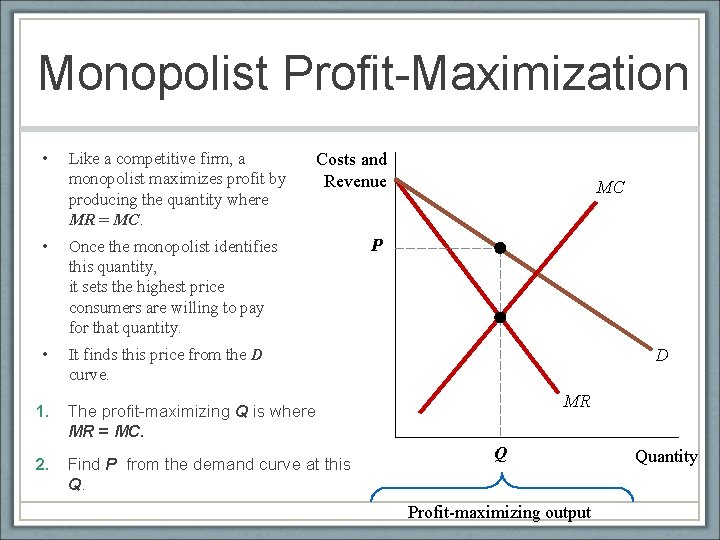Monopolist Profit-Maximization • Like a competitive firm, a monopolist maximizes profit by producing the quantity where MR = MC. • Once the monopolist identifies this quantity, it sets the highest price consumers are willing to pay for that quantity. • It finds this price from the D curve. Costs and Revenue 1. The profit-maximizing Q is where MR = MC. 2. Find P from the demand curve at this Q. MC P D MR Q Profit-maximizing output QuantityA Monopoly Does Not Have a Supply Curve! A competitive firm • takes P as given • has a supply curve that shows how its Q depends on P. A monopoly firm • is a “price-maker, ” not a “price-taker” • Q does not depend on P; rather, Q and P are jointly determined by MC, MR, and the demand curve. So there is no supply curve for monopoly.4. Monopoly, welfare, and public policyThe Welfare Cost of Monopoly • Recall: In a competitive market equilibrium, P = MC and total surplus is maximized. • In the monopoly equilibrium, P > MR = MC • The value to buyers of an additional unit (P) exceeds the cost of the resources needed to produce that unit (MC). • The monopoly Q is too low – could increase total surplus with a larger Q. • Thus, monopoly results in a deadweight loss.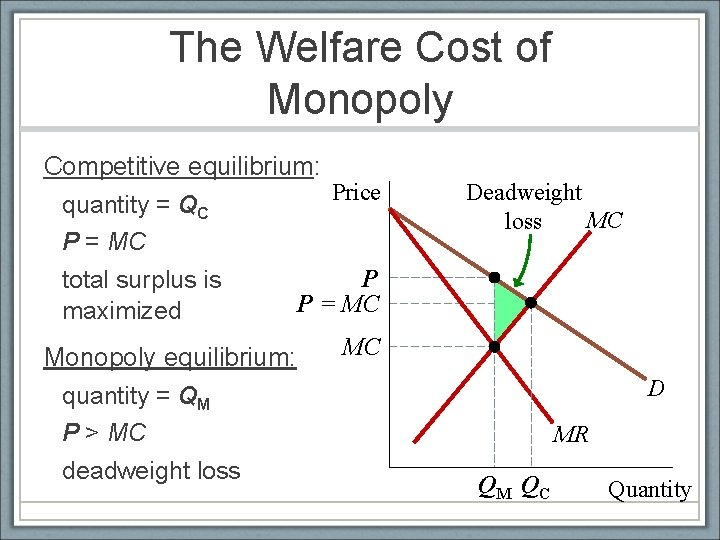The Welfare Cost of Monopoly Competitive equilibrium: Price quantity = QC P = MC P total surplus is P = MC maximized MC Monopoly equilibrium: quantity = QM P > MC deadweight loss Deadweight MC loss D MR QM QC QuantityIn comparison to a perfectly competitive firm, a monopolist charges a 1. higher price and produces a higher quantity. 2. higher price and produces a lower quantity. 3. lower price and produces a higher quantity. 4. lower price and produces a lower quantity.Refer to the figure below. The deadweight loss for a profit-maximizing monopolist is the area 1. ABEG. 3. FBE. 2. ABC. 4. GEC.Public Policy Toward Monopolies • Increasing competition with antitrust laws • Ban some anticompetitive practices, allows government to break up monopolies. • Regulation • Government agencies set the monopolist’s price. • Public ownership • Example: U. S. Postal Service • Problem: Public ownership is usually less efficient since no profit motive to minimize costs • Doing nothing? • The foregoing policies all have drawbacks, so the best policy may be no policy (if costs exceed benefits)